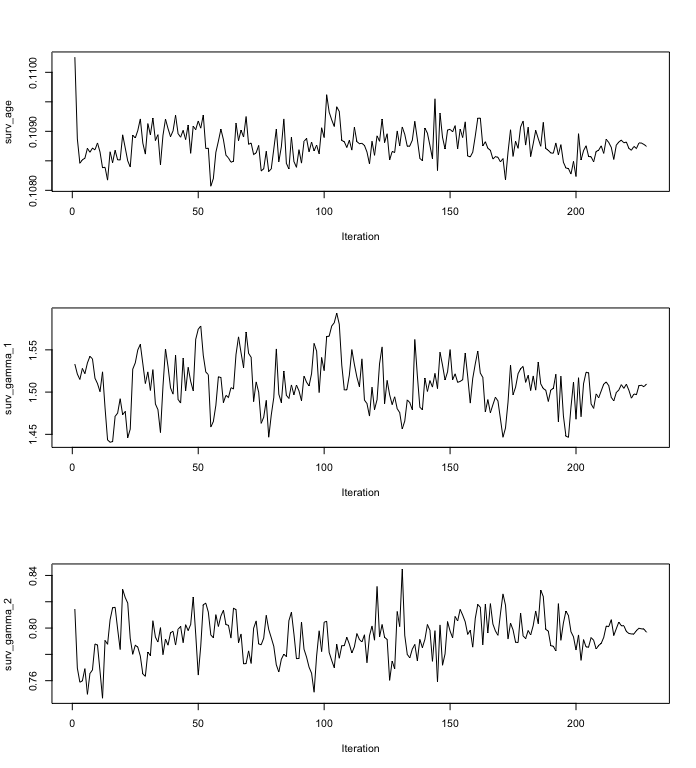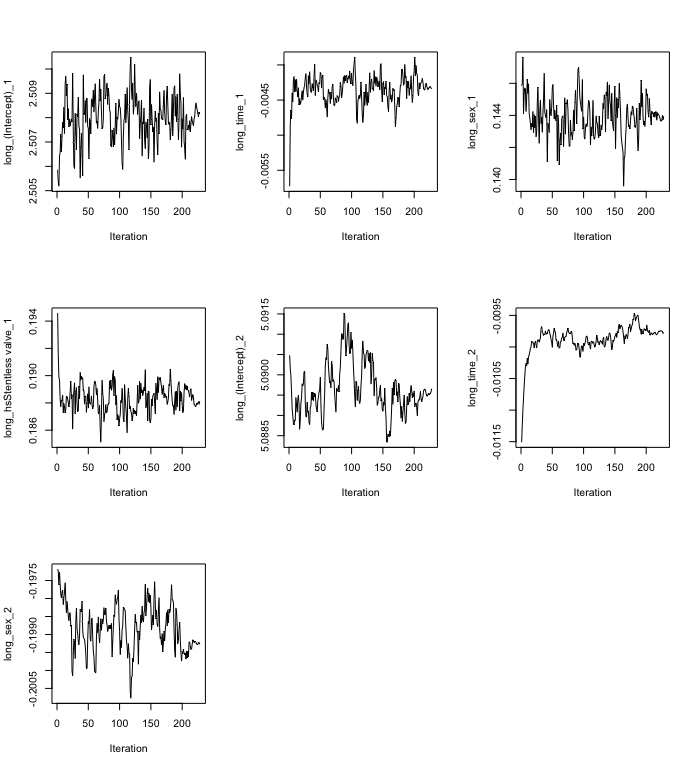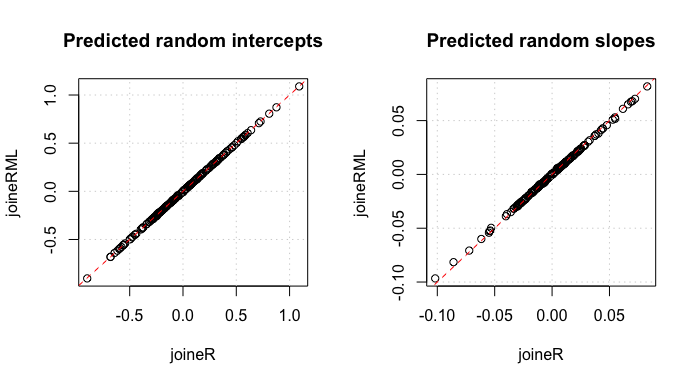Introduction

The joineRML package implements methods for analyzing data from multiple longitudinal studies in which the responses from each subject consists of time-sequences of repeated measurements and a possibly censored time-to-event outcome. The modelling framework for the repeated measurements is the multivariate linear mixed effects model. The model for the time-to-event outcome is a Cox proportional hazards model with log-Gaussian frailty. Stochastic dependence is captured by allowing the Gaussian random effects of the linear model to be correlated with the frailty term of the Cox proportional hazards model. For full details of the model, please consult the technical vignette by running

vignette("technical", package = "joineRML")

Heart valve data

Data

The simplest way to explain the concepts of the package is through an example. joineRML comes with the data set heart.valve. Details of this data can be found in the help file by running the command

help("heart.valve", package = "joineRML")

This data is in so-called long or unbalanced format:

library("joineRML")
data("heart.valve")
##   num sex      age      time    fuyrs status grad log.grad   lvmi log.lvmi
## 1   1   0 75.06027 0.0109589 4.956164      0   10 2.302585 118.98 4.778955
## 2   1   0 75.06027 3.6794520 4.956164      0   10 2.302585 118.98 4.778955
## 3   1   0 75.06027 4.6958900 4.956164      0   10 2.302585 137.63 4.924569
## 4   2   0 45.79452 6.3643840 9.663014      0   14 2.639057 114.93 4.744323
## 5   2   0 45.79452 7.3041100 9.663014      0    9 2.197225 109.80 4.698661
## 6   2   0 45.79452 8.3013700 9.663014      0   12 2.484907 157.40 5.058790
##   ef  bsa lvh prenyha redo size con.cabg creat dm acei lv emergenc hc
## 1 93 1.77   1       3    0   27        1   103  0    1  1        0  0
## 2 93 1.77   1       3    0   27        1   103  0    1  1        0  0
## 3 93 1.77   1       3    0   27        1   103  0    1  1        0  0
## 4 68 1.92   1       1    1   22        0    76  0    0  2        0  0
## 5 70 1.92   1       1    1   22        0    76  0    0  2        0  0
## 6 56 1.92   1       1    1   22        0    76  0    0  2        0  0
##   sten.reg.mix              hs
## 1            1 Stentless valve
## 2            1 Stentless valve
## 3            1 Stentless valve
## 4            1       Homograft
## 5            1       Homograft
## 6            1       Homograft

The data refer to 256 patients and are stored in the unbalanced format, which is convenient here because measurement times were unique to each subject. The data are stored as a single R object, heart.valve, which is a data frame of dimension 988 by 25. The average number of repeated measurements per subject is therefore 988/256 = 3.86. As with any unbalanced data set, values of time-constant variables are repeated over all rows that refer to the same subject. The dimensionality of the data set can be confirmed by a call to the dim() function, whilst the names of the 25 variables can be listed by a call to the names() function:

dim(heart.valve)
##  988  25
names(heart.valve)
##   "num"          "sex"          "age"          "time"
##   "lvmi"         "log.lvmi"     "ef"           "bsa"
##  "lvh"          "prenyha"      "redo"         "size"
##  "con.cabg"     "creat"        "dm"           "acei"
##  "lv"           "emergenc"     "hc"           "sten.reg.mix"
##  "hs"

We will only analyse a subset of this data, namely records with case-complete data for heart valve gradient (grad) and left ventricular mass index (lvmi):

hvd <- heart.valve[!is.na(heart.valve$grad) & !is.na(heart.valve$lvmi), ]

Strictly speaking, this is not necessary because joineRML can handle the situation of different measurement schedules within subjects That is, a subject does not need to have all multiple longitudinal outcomes recorded at each visit. It is conceivable that some biomarkers will be measured more or less frequently than others. For example, invasive measurements may only be recorded annually, whereas a simple biomarker measurement might be recorded more frequently. joineRML can handle this situation by specifying each longitudinal outcome its own data frame.

Model fitting

The main function in the joineRML package is the mjoint() function. Its main (required) arguments are:

• formLongFixed: a list (of length equal to the number of longitudinal outcome types considered) of two-sided formulae specifying the response on the left-hand side and the mean linear predictor terms for the fixed effects in the linear mixed models on the right-hand side.

• formLongRandom: a list (of same length as formLongFixed) of one-sided formulae specifying the model for random effects in the linear mixed models.

• formSurv: a formula specifying the proportional hazards regression model for the time-to-event outcome in the same structure as for survival::coxph.

• data: a list (of same length as formLongFixed) of data.frames; one for each longitudinal outcome. It is assumed that the event time data is in the first data.frame (i.e. data[]), unless the argument survData (which defaults to NULL) is specified. If $$K>1$$ and the data are balanced within patients (i.e. multiple markers measured at common measurement times), then one can specify data as a data frame rather than as a list.

• timeVar: the column name indicating the time variable in the linear mixed effects model. If $$K>1$$ and the data frames have different column names for time, then timeVar can alternatively be specified as a vector of strings of length $$K$$.

We can fit a bivariate joint model to the log-transformed valve gradient and LVMI indices in the hvd subset using

set.seed(12345)
fit <- mjoint(
formLongFixed = list("grad" = log.grad ~ time + sex + hs,
"lvmi" = log.lvmi ~ time + sex),
formLongRandom = list("grad" = ~ 1 | num,
"lvmi" = ~ time | num),
formSurv = Surv(fuyrs, status) ~ age,
data = list(hvd, hvd),
inits = list("gamma" = c(0.11, 1.51, 0.80)),
timeVar = "time")
## Running multivariate LMM EM algorithm to establish initial parameters...
## Finished multivariate LMM EM algorithm...
## EM algorithm has converged!
## Calculating post model fit statistics...

Details on the model estimation algorithm are provided in the technical details vignette. We note here that this is not necessarily the most appropriate model for the data, and is included only for the purposes of demonstration. There are a number of other useful arguments in the mjoint function; for example, inits for specifying (partial) initial values, control for controlling the optimization algorithm, and verbose for monitoring the convergence output in real-time. A full list of all arguments with explanation are given in the help documentation, accessed by running help("mjoint").

Post-fit analysis

Once we have a fitted mjoint object, we can begin to extract relevant information from it. Most summary statistics are available from the summary function:

summary(fit)
##
## Call:
## mjoint(formLongFixed = list(grad = log.grad ~ time + sex + hs,
##     lvmi = log.lvmi ~ time + sex), formLongRandom = list(grad = ~1 |
##     num, lvmi = ~time | num), formSurv = Surv(fuyrs, status) ~
##     age, data = list(hvd, hvd), timeVar = "time", inits = list(gamma = c(0.11,
##     1.51, 0.8)))
##
## Data Descriptives:
##
## Event Process
##     Number of subjects: 221
##     Number of events: 47 (21.3%)
##
## Longitudinal Process
##     Number of longitudinal outcomes: K = 2
##     Number of observations:
##       Outcome 1 (grad): n = 629
##       Outcome 2 (lvmi): n = 629
##
## Joint Model Summary:
##
## Longitudinal Process: Multivariate linear mixed-effects model
##      log.grad ~ time + sex + hs, random = ~1 | num
##      log.lvmi ~ time + sex, random = ~time | num
## Event Process: Cox proportional hazards model
##      Surv(fuyrs, status) ~ age
## Model fit statistics:
##    log.Lik      AIC      BIC
##  -991.1137 2018.227 2079.394
##
## Variance Components:
##
## Random effects variance covariance matrix
##               (Intercept)_1 (Intercept)_2     time_2
## (Intercept)_1     0.1058000      0.019288  0.0036944
## (Intercept)_2     0.0192880      0.118970 -0.0062780
## time_2            0.0036944     -0.006278  0.0024684
##   Standard Deviations: 0.32527 0.34492 0.049683
##
## Residual standard errors(s):
##  sigma2_1  sigma2_2
## 0.5961137 0.1927721
##
## Coefficient Estimates:
##
## Longitudinal sub-model:
##                       Value Std.Err  z-value p-value
## (Intercept)_1        2.5082  0.0642  39.0944 <0.0001
## time_1              -0.0043  0.0133  -0.3256  0.7447
## sex_1                0.1439  0.0769   1.8707  0.0614
## hsStentless valve_1  0.1881  0.0743   2.5321  0.0113
## (Intercept)_2        5.0897  0.0339 150.2164 <0.0001
## time_2              -0.0098  0.0068  -1.4363  0.1509
## sex_2               -0.1993  0.0549  -3.6271  0.0003
##
## Time-to-event sub-model:
##          Value Std.Err z-value p-value
## age     0.1087  0.0160  6.7832 <0.0001
## gamma_1 1.5097  0.9038  1.6704  0.0948
## gamma_2 0.7968  0.6469  1.2316  0.2181
##
## Algorithm Summary:
##     Total computational time: 1.2 mins
##     EM algorithm computational time: NA mins
##     Convergence status: converged
##     Convergence criterion: sas
##     Final Monte Carlo sample size: 5549
##     Standard errors calculated using method: approx

One can also extract the coefficients, fixed effects, and random effects using standard generic functions:

coef(fit)
## $D ## (Intercept)_1 (Intercept)_2 time_2 ## (Intercept)_1 0.105802296 0.019288342 0.003694426 ## (Intercept)_2 0.019288342 0.118969721 -0.006277957 ## time_2 0.003694426 -0.006277957 0.002468353 ## ##$beta
##       (Intercept)_1              time_1               sex_1
##         2.508158052        -0.004341931         0.143942569
## hsStentless valve_1       (Intercept)_2              time_2
##         0.188133752         5.089659402        -0.009781482
##               sex_2
##        -0.199276836
##
## $sigma2 ## sigma2_1 sigma2_2 ## 0.35535159 0.03716109 ## ##$haz
##   0.001867911 0.001875488 0.002019183 0.002227192 0.002262268
##   0.002339430 0.002396734 0.002400211 0.002438572 0.002454757
##  0.002468868 0.002677928 0.002733153 0.002791940 0.002812594
##  0.002847601 0.002897962 0.003033319 0.003165194 0.003371493
##  0.003654905 0.007654983 0.004255871 0.004474953 0.004580072
##  0.004772205 0.005185041 0.005314130 0.006236632 0.006772426
##  0.006941574 0.007154566 0.007266036 0.007859618 0.008533176
##  0.008897458 0.009259170 0.010058941 0.011949178 0.012322053
##  0.013659672 0.016515838 0.017034043 0.046464128 0.048604005
##  0.247706507
##
## $gamma ## age gamma_1 gamma_2 ## 0.1087459 1.5096743 0.7967613 fixef(fit, process = "Longitudinal") ## (Intercept)_1 time_1 sex_1 ## 2.508158052 -0.004341931 0.143942569 ## hsStentless valve_1 (Intercept)_2 time_2 ## 0.188133752 5.089659402 -0.009781482 ## sex_2 ## -0.199276836 fixef(fit, process = "Event") ## age gamma_1 gamma_2 ## 0.1087459 1.5096743 0.7967613 head(ranef(fit)) ## (Intercept)_1 (Intercept)_2 time_2 ## 1 -0.20321013 -0.244778995 0.010441871 ## 2 -0.04384301 -0.176901441 0.001807459 ## 3 -0.01740527 0.006699592 0.010510071 ## 4 -0.42728153 -0.596236132 0.012313738 ## 5 -0.05173721 0.077815662 0.021615134 ## 6 0.23306568 0.227851417 0.006021618 Although a model fit may indicate convergence, it is generally a good idea to examine the convergence plots. These can be viewed using the plot function for each group of model parameters. plot(fit, params = "gamma")plot(fit, params = "beta")Bootstrap standard errors Once an mjoint model has converged, and assuming the pfs argument is TRUE (default), then approximated standard errors are calculated based on the empirical information matrix of the profile likelihood at the maximizer. Theoretically, these standard errors will be underestimated (see the technical vignette). In principle, residual Monte Carlo error will oppose this through an increase in uncertainty. fit.se <- bootSE(fit, nboot = 100) Bootstrapping is a computationally intensive method, possibly taking many hours to fit. For this reason, one can relax the control parameter constraints on the optimization algorithm for each bootstrap model; however, this will be at the possible expense of inflated standard errors due to Monte Carlo error. We can call the bootSE object to interrogate it fit.se or alternatively re-run the summary command, passing the additional argument of bootSE = fit.se summary(fit, bootSE = fit.se) Univariate joint models: joineRML versus joineR There are a growing number of software options for fitting joint models of a single longitudinal outcome and a single time-to-event outcome; what we call here univariate joint models. joineR (version 1.1.0) is one package available in R for fitting such models, however joineRML can fit these models too, since the univariate model is simply a special case of the multivariate model. It is useful to contrast these two packages. There are theoretical and practical implementation differences between the packages beyond just univariate versus multivariate capability: • joineR uses Gauss-Hermite quadrature (with 3 nodes) for numerical integration, whereas joineRML uses Monte Carlo integration with an automated selection of sample size. • joineR only allows for random-intercept models, random-intercept and random-slope models, or a quadratic model. joineRML, on the other hand, allows for any random effects structure. • joineR only allows for specification of convergence based on an absolute difference criterion. • joineR does not calculate approximate standard errors, and instead requires a bootstrap approach be used after the model fit. • The current version of joineR requires a data pre-processing step in order to generate a joint.data object, whereas joineRML can work straight from the data frame. To fit a univariate model in joineR we run the following code for the hvd data library(joineR, quietly = TRUE) hvd.surv <- UniqueVariables(hvd, var.col = c("fuyrs", "status"), id.col = "num") hvd.cov <- UniqueVariables(hvd, "age", id.col = "num") hvd.long <- hvd[, c("num", "time", "log.lvmi")] hvd.jd <- jointdata(longitudinal = hvd.long, baseline = hvd.cov, survival = hvd.surv, id.col = "num", time.col = "time") fit.joiner <- joint(data = hvd.jd, long.formula = log.lvmi ~ time + age, surv.formula = Surv(fuyrs, status) ~ age, model = "intslope") summary(fit.joiner) ## ## Call: ## joint(data = hvd.jd, long.formula = log.lvmi ~ time + age, surv.formula = Surv(fuyrs, ## status) ~ age, model = "intslope") ## ## Random effects joint model ## Data: hvd.jd ## Log-likelihood: -373.2656 ## ## Longitudinal sub-model fixed effects: log.lvmi ~ time + age ## (Intercept) 4.9979864721 ## time -0.0096970070 ## age 0.0004407409 ## ## Survival sub-model fixed effects: Surv(fuyrs, status) ~ age ## age 0.1058821 ## ## Latent association: ## gamma_0 1.072406 ## ## Variance components: ## U_0 U_1 Residual ## 0.128470783 0.002561009 0.037055051 ## ## Convergence at iteration: 18 ## ## Number of observations: 629 ## Number of groups: 221 To fit a univariate model in joineRML we run the following code for the hvd data set.seed(123) fit.joinerml <- mjoint(formLongFixed = log.lvmi ~ time + age, formLongRandom = ~ time | num, formSurv = Surv(fuyrs, status) ~ age, data = hvd, timeVar = "time") ## EM algorithm has converged! ## Calculating post model fit statistics... summary(fit.joinerml) ## ## Call: ## mjoint(formLongFixed = log.lvmi ~ time + age, formLongRandom = ~time | ## num, formSurv = Surv(fuyrs, status) ~ age, data = hvd, timeVar = "time") ## ## Data Descriptives: ## ## Event Process ## Number of subjects: 221 ## Number of events: 47 (21.3%) ## ## Longitudinal Process ## Number of longitudinal outcomes: K = 1 ## Number of observations: ## Outcome 1: n = 629 ## ## Joint Model Summary: ## ## Longitudinal Process: Univariate linear mixed-effects model ## log.lvmi ~ time + age, random = ~time | num ## Event Process: Cox proportional hazards model ## Surv(fuyrs, status) ~ age ## Model fit statistics: ## log.Lik AIC BIC ## -373.2707 764.5414 795.1249 ## ## Variance Components: ## ## Random effects variance covariance matrix ## (Intercept)_1 time_1 ## (Intercept)_1 0.1277800 -0.0065414 ## time_1 -0.0065414 0.0024152 ## Standard Deviations: 0.35746 0.049145 ## ## Residual standard errors: ## sigma2_1 ## 0.193006 ## ## Coefficient Estimates: ## ## Longitudinal sub-model: ## Value Std.Err z-value p-value ## (Intercept)_1 4.9983 0.1401 35.6856 <0.0001 ## time_1 -0.0093 0.0067 -1.4049 0.1600 ## age_1 0.0004 0.0021 0.2069 0.8361 ## ## Time-to-event sub-model: ## Value Std.Err z-value p-value ## age 0.1060 0.0149 7.0969 <0.0001 ## gamma_1 1.0846 0.5413 2.0036 0.0451 ## ## Algorithm Summary: ## EM algorithm computational time: 10.1 secs ## Convergence status: converged ## Convergence criterion: sas ## Final Monte Carlo sample size: 418 ## Standard errors calculated using method: approx In addition to just comparing model parameter estimates, we can also extract the predicted (or posterior) random effects from each model and plot them. id <- as.numeric(row.names(fit.joiner$coefficients$random)) id.ord <- order(id) # joineR rearranges patient ordering during EM fit par(mfrow = c(1, 2)) plot(fit.joiner$coefficients$random[id.ord, 1], ranef(fit.joinerml)[, 1], main = "Predicted random intercepts", xlab = "joineR", ylab = "joineRML") grid() abline(a = 0, b = 1, col = 2, lty = "dashed") plot(fit.joiner$coefficients\$random[id.ord, 2], ranef(fit.joinerml)[, 2],
main = "Predicted random slopes",
xlab = "joineR", ylab = "joineRML")
grid()
abline(a = 0, b = 1, col = 2, lty = "dashed")